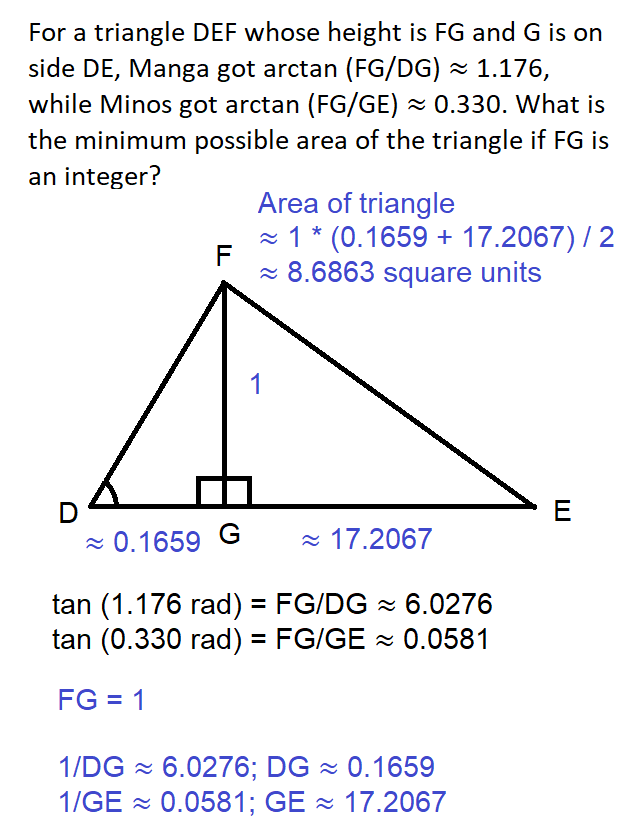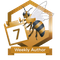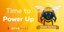# Result of the Day 5 Challenge of my May 2021 Math trigonometry mini-contest for D.Buzz 😎

in STEMGeeks2 months ago

## For a triangle DEF whose height is FG and G is on side DE, Manga got `arctan (FG/DG) ≈ 1.176`, while Minos got `arctan (FG/GE) ≈ 0.330`. What is the minimum possible area of the triangle if FG is an integer?

The problem was originally published at https://stemgeeks.net/@savvyplayer/mayday5math.

# 8.6863 square units 🎯

## SolutionSorry again for the gritty graphics, because I still use MS Paint to draw the image above! 😅

The given states the following:

• arctan (FG/DG) ≈ 1.176 radians
• arctan (FG/GE) ≈ 0.330 radians
• FG is integer
• DE = DG + GE

Based on the given facts above, we can derive the following facts:

• FG/DG = tan (1.176) ≈ 6.0276, so FG ≈ 6.0276 * DG, which gives us DG ≈ 0.1659
• FG/GE = tan (0.330) ≈ 0.0581, so FG ≈ 0.0581 * GE, which gives us GE ≈ 17.2067
• The smaller FG is, the smaller the area of the triangle will be, regardless of the measure of the other sides of the triangle. Therefore, we should set it to 1.
• Since we have assumed FG to be 1, DG * 6.0276 ≈ 1 and GE * 0.0581 ≈ 1, which gives us DG ≈ 0.1659 and GE ≈ 17.2067
• Since DE = DG + GE, DE = 0.1659 + 17.2067 ≈ 17.3727

To get the area of the triangle, we just need its base length and height which are DE and FG, respectively, which we already derived. `area = (DE + FG) / 2 ≈ (17.3727 + 1) / 2 ≈ 8.6863 square units`.

# Winner: none 🤯

Today's prize of 1 HIVE has been split depending on the number of winners in the previous days for this month's contest and sent to the only winning participant so far for this month's Math mini-contest for D.Buzz, which is still @minus-pi! 💰😅

• @minus-pi forgot to add the "square units" again on his answer! 🤯😅

Special mentions: @dbuzz, @chrisrice, @jancharlest, and @mehmetfix 🤯

Posted with STEMGeeks

Sort:

lol, again, same mistake 😅
thanks anyway!

Maybe if I was able to show that day's solution quickly after the 24-hour answering period for the Day 4 Math problem, you would have changed your answer for the Day 5 Problem! 🤔😁

Congratulations @savvyplayer! You have completed the following achievement on the Hive blockchain and have been rewarded with new badge(s) :You have been a buzzy bee and published a post every day of the week

If you no longer want to receive notifications, reply to this comment with the word `STOP`Hive Power Up Day - June 1st 2021 - Hive Power Delegation